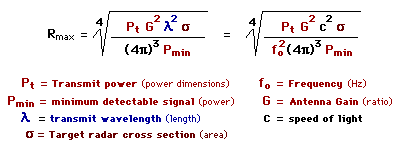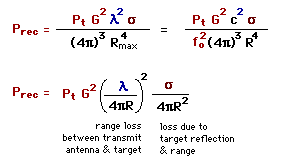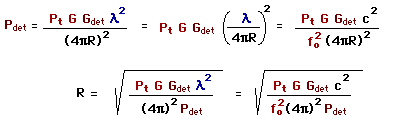Home

Page Outline

There exist hundreds of versions of the radar range equation. Below is one of the more basic forms for a single antenna system (same antenna for both transmit and receive). The target is assumed to be in the center of the antenna beam. The maximum radar detection range is;The variables in the above equation are constant and radar dependent except target RCS. Transmit power will be on the order of 1 mW (0 dBm) and antenna gain around 100 (20 dB) for an effective radiated power (ERP) of 100 mW (20 dBm). Minimum detectable signals are on the order of picowatts; RCS for an automobile might be on the order of 100 square meters. The accuracy of the radar range equation is only as good as the input data.

Minimum detectable signal (Pmin) depends on receiver bandwidth (B), noise figure (F), temperature (T), and required signal-to-noise ratio (S/N). A narrow bandwidth receiver will be more sensitive than a wider bandwidth receiver. Noise figure is a measure of how much noise a device (the receiver) contributes to a signal: the smaller the noise figure, the less noise the device contributes. Increasing temperature affects receiver sensitivity by increasing input noise.
 Pmin = k T B F (S/N)min
Pmin = Minimum Detectable Signal
k = Blotzmann's Constant = 1.38 x 10-23 (Watt*sec/°Kelvin)
T = Temperature (°Kelvin)
F = Noise Factor (ratio), Noise Figure (dB)
(S/N)min = Minimum Signal to Noise Ratio

The available input thermal noise power (background noise) is proportional to the product kTB where k is Boltzmann's constant, T is temperature (degrees Kelvin) and B is receiver noise bandwidth (approximately receiver bandwidth) in hertz.
T = 290°K (62.33°F), B = 1 Hz
 kTB = -174 dBm/Hz
The radar range equation above can be written for power received as a function of range for a given transmit power, wavelength, antenna gain, and RCS.Prec = Power Received Pt = Transmit Power fo = Transmit Frequency Lamda = Transmit Wavelength G = Antenna Gain Sigma = Radar Cross Section R = Range c = Speed of Light